# C# Program For Deleting A Node In A Doubly Linked List

• Last Updated : 15 Dec, 2021

Pre-requisite: Doubly Link List Set 1| Introduction and Insertion

Write a function to delete a given node in a doubly-linked list.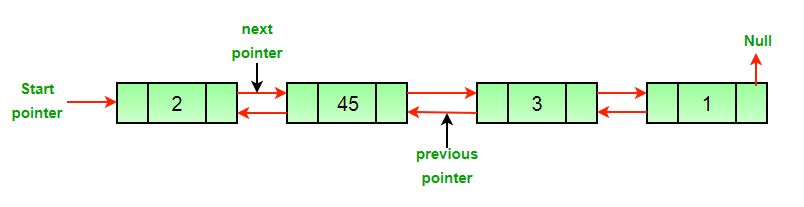Approach: The deletion of a node in a doubly-linked list can be divided into three main categories:

• After the deletion of the head node.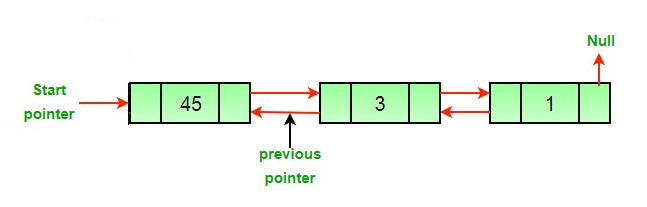• After the deletion of the middle node.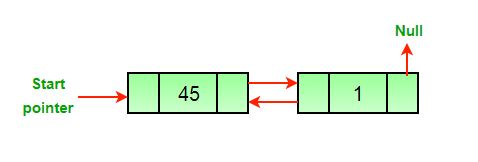• After the deletion of the last node.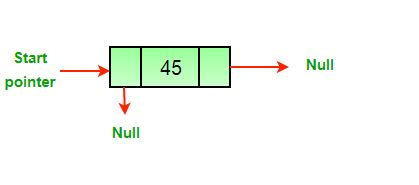All three mentioned cases can be handled in two steps if the pointer of the node to be deleted and the head pointer is known.

1. If the node to be deleted is the head node then make the next node as head.
2. If a node is deleted, connect the next and previous node of the deleted node.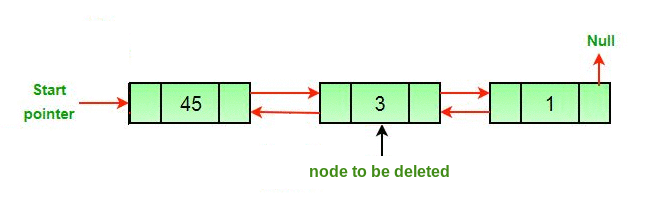Algorithm

• Let the node to be deleted be del.
• If node to be deleted is head node, then change the head pointer to next current head.
```if headnode == del then
• Set next of previous to del, if previous to del exists.
```if del.nextNode != none
del.nextNode.previousNode = del.previousNode ```
• Set prev of next to del, if next to del exists.
```if del.previousNode != none
del.previousNode.nextNode = del.next```

## C#

 `// C# program to delete a node from``// Doubly Linked List``using` `System;`` ` `// Class for Doubly Linked List``public` `class` `DLL ``{``    ``// head of list``    ``Node head; `` ` `    ``// Doubly Linked list Node``    ``public` `class` `Node ``    ``{``        ``public` `int` `data;``        ``public` `Node prev;``        ``public` `Node next;`` ` `        ``// Constructor to create a new node``        ``// next and prev is by default ``        ``// initialized as null``        ``public` `Node(``int` `d) ``        ``{ ``            ``data = d; ``        ``}``    ``}`` ` `    ``// Adding a node at the front of ``    ``// the list``    ``public` `void` `push(``int` `new_data)``    ``{``        ``// 1. Allocate node``        ``// 2. Put in the data``        ``Node new_Node = ``new` `Node(new_data);`` ` `        ``// 3. Make next of new node as head``        ``//    and previous as NULL``        ``new_Node.next = head;``        ``new_Node.prev = ``null``;`` ` `        ``// 4. Change prev of head node to ``        ``//    new node``        ``if` `(head != ``null``)``            ``head.prev = new_Node;`` ` `        ``// 5. Move the head to point to ``        ``//    the new node``        ``head = new_Node;``    ``}`` ` `    ``// This function prints contents of ``    ``// linked list starting from the ``    ``// given node``    ``public` `void` `printlist(Node node)``    ``{`` ` `        ``while` `(node != ``null``)``        ``{``            ``Console.Write(node.data + ``" "``);``            ``node = node.next;``        ``}`` ` `        ``Console.WriteLine();``    ``}`` ` `    ``// Function to delete a node in a Doubly ``    ``// Linked List. head_ref --> pointer to ``    ``// head node pointer. del --> data of node ``    ``// to be deleted.``    ``void` `deleteNode(Node del)``    ``{``        ``// Base case``        ``if` `(head == ``null` `|| del == ``null``) ``        ``{``            ``return``;``        ``}`` ` `        ``// If node to be deleted is head node``        ``if` `(head == del) ``        ``{``            ``head = del.next;``        ``}`` ` `        ``// Change next only if node to ``        ``// be deleted is NOT the last node``        ``if` `(del.next != ``null``) ``        ``{``            ``del.next.prev = del.prev;``        ``}`` ` `        ``// Change prev only if node to be ``        ``// deleted is NOT the first node``        ``if` `(del.prev != ``null``) ``        ``{``            ``del.prev.next = del.next;``        ``}`` ` `        ``// Finally, free the memory occupied ``        ``// by del``        ``return``;``    ``}`` ` `    ``// Driver Code``    ``public` `static` `void` `Main()``    ``{``        ``// Start with the empty list``        ``DLL dll = ``new` `DLL();`` ` `        ``// Insert 2. So linked list ``        ``// becomes 2->NULL``        ``dll.push(2);`` ` `        ``// Insert 4. So linked list ``        ``// becomes 4->2->NULL``        ``dll.push(4);`` ` `        ``// Insert 8. So linked list ``        ``// becomes 8->4->2->NULL``        ``dll.push(8);`` ` `        ``// Insert 10. So linked list ``        ``// becomes 10->8->4->2->NULL``        ``dll.push(10);`` ` `        ``Console.Write(``"Created DLL is: "``);``        ``dll.printlist(dll.head);`` ` `        ``// Deleting first node``        ``dll.deleteNode(dll.head);`` ` `        ``// List after deleting first node``        ``// 8->4->2``        ``Console.Write(``        ``"List after deleting first node: "``);``        ``dll.printlist(dll.head);`` ` `        ``// Deleting middle node from 8->4->2``        ``dll.deleteNode(dll.head.next);`` ` `        ``Console.Write(``        ``"List after Deleting middle node: "``);``        ``dll.printlist(dll.head);``    ``}``}``// This code is contributed by PrinciRaj1992`

Output:

```Original Linked list 10 8 4 2

Complexity Analysis:

• Time Complexity: O(1).
Since traversal of the linked list is not required so the time complexity is constant.
• Space Complexity: O(1).
As no extra space is required, so the space complexity is constant.

Please refer complete article on Delete a node in a Doubly Linked List for more details!

My Personal Notes arrow_drop_up